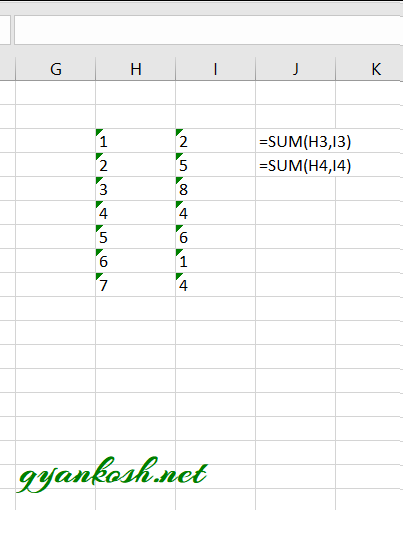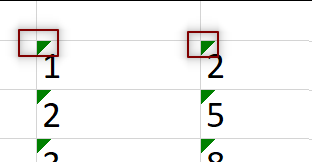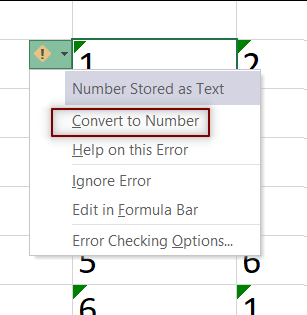PROVIDING ACCURATE, RELIABLE AND STRAIGHT TO THE POINT KNOWLEDGE ABOUT DIFFERENT IT TOOLS EXPLAINED WITH PRACTICAL EXAMPLES

# HOW TO SOLVE NUMBER STORED AS TEXT PROBLEM

## INTRODUCTION

Whenever we prepare any report in excel, we have two constituents in any report.

The Text portion and the Numerical portion.

But just storing the text and numbers doesn’t make the super reports. Many times we need to automate the process in the reports to minimize the effort and improve the accuracy.

Many functions are provided by the Excel which work on Text and give us the useful output as well. But few problems are still left on which we need to apply some tricks with the available tools.

In this articles , we are going to learn to get rid of the problem called NUMBER STORED AS TEXT.

## HOW TEXT IS HANDLED IN EXCEL?

TEXT is simply the group of characters and strings of characters which convey the information about the different data and numbers in Excel. Every character is connected with a code [ANSI].
Text comprises of the individual entity character which is the smallest bit which would be found in Excel.
We can perform the operations on the strings[Text] or the characters.
Characters are not limited to A to Z or a to z but many symbols are also included in this which we would see in the later part of the article.

TEXT IS AN INACTIVE NUMBER TYPE[FORMAT] IN EXCEL. ANYTHING STORED AS TEXT [NUMBER OR DATE] WON’T RESPOND TO ANY STANDARD FORMULAS OR FUNCTIONS BUT SPECIALLY DESIGNED TEXT FUNCTIONS. [EXCEPTIONS DO OCCUR IN CASE OF NUMBERS]
If we need to make anything inactive, such as Date to be non responding to the calculation, we put it as a text. Similarly if we want to avoid any calculations for a number it needs to be put as a text.

## NUMBER STORED AS TEXT PROBLEM IN EXCEL

### CONCEPT:

Have you ever noticed that your cell contain a number [ visibly number] but it is not responding to your formulas or functions as expected. Most probably it is because of the format of the cell or NUMBER TYPE OF THE CELL.
Out of many problems with the formatting of the cell , we are going to focus on the one called NUMBER STORED AS TEXT.
Sometimes or we say most of the time when we copy any number from somewhere or get the data through any channel, we get this problem that the NUMBER IS VISIBLE AS A NUMBER BUT THE FORMAT IS TEXT. The problem with such numbers is that they won’t respond to our calculations. Look at the picture below.As we can see from the picture above that the text is not responding to the functions. [Although Excel is smart enough to do the simple tasks like addition , subtraction etc. even with the number stored as text]

### HOW TO RECOGNIZE IF THE NUMBER IS STORED AS TEXT?

That is pretty simple. Excel itself marks it by a small TRIANGLE AT THE LEFT UPPER CORNER.### HOW TO CONVERT NUMBER STORED AS TEXT , BACK TO NUMBER?

Just click the TRIANGLE MENTIONED ABOVE.Excel will give you a number of options like• ## WHAT IS EXCEL, HOW EXCEL WORKS, WRITE FORMULA IN EXCEL, EXAMPLE OF USING FORMULA IN EXCEL, ANIMATED EXAMLPE OF USING FORMULA IN EXCEL

LEARN

• EXCEL
• JAVASCRIPT
• MORE TO COME…

Encourage us by following at …

If you have any queries or suggestions or any problem which you would like us to solve for you, kindly mail at

[email protected]